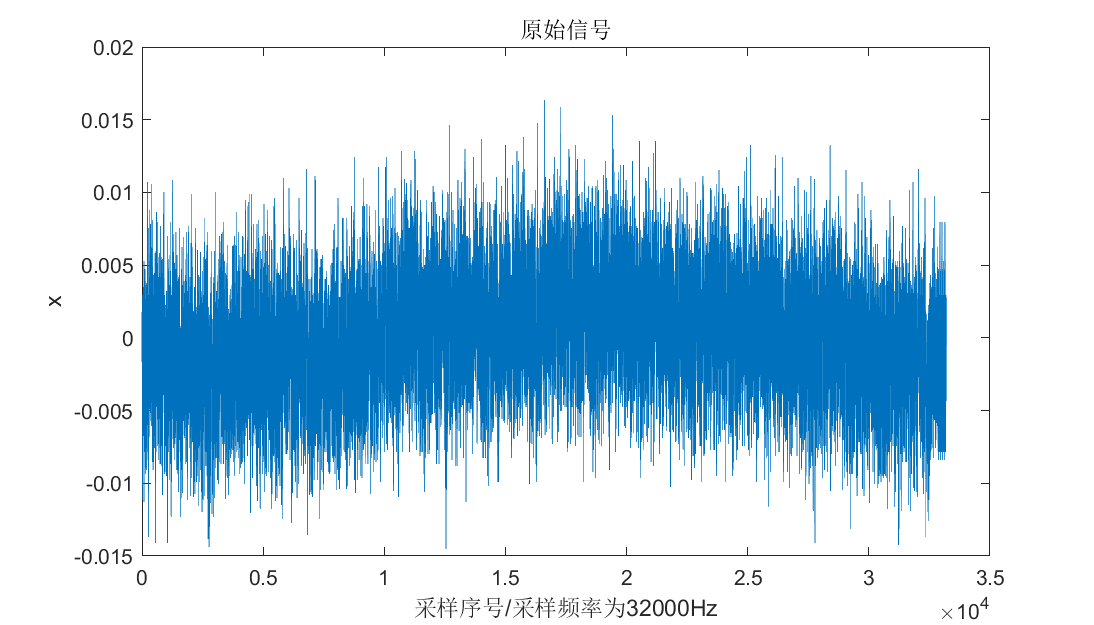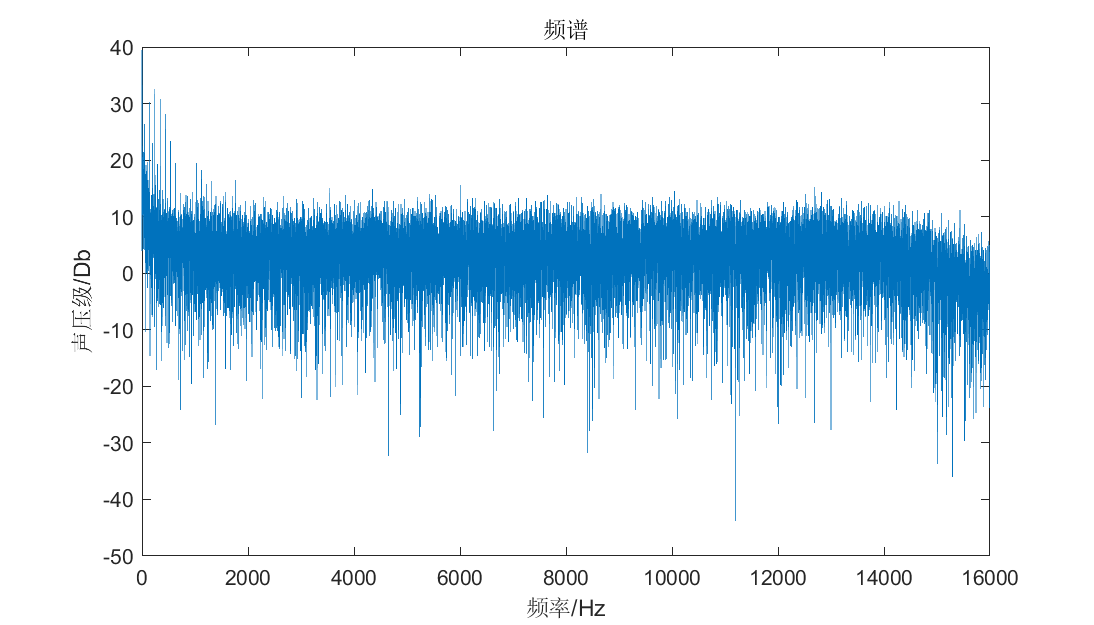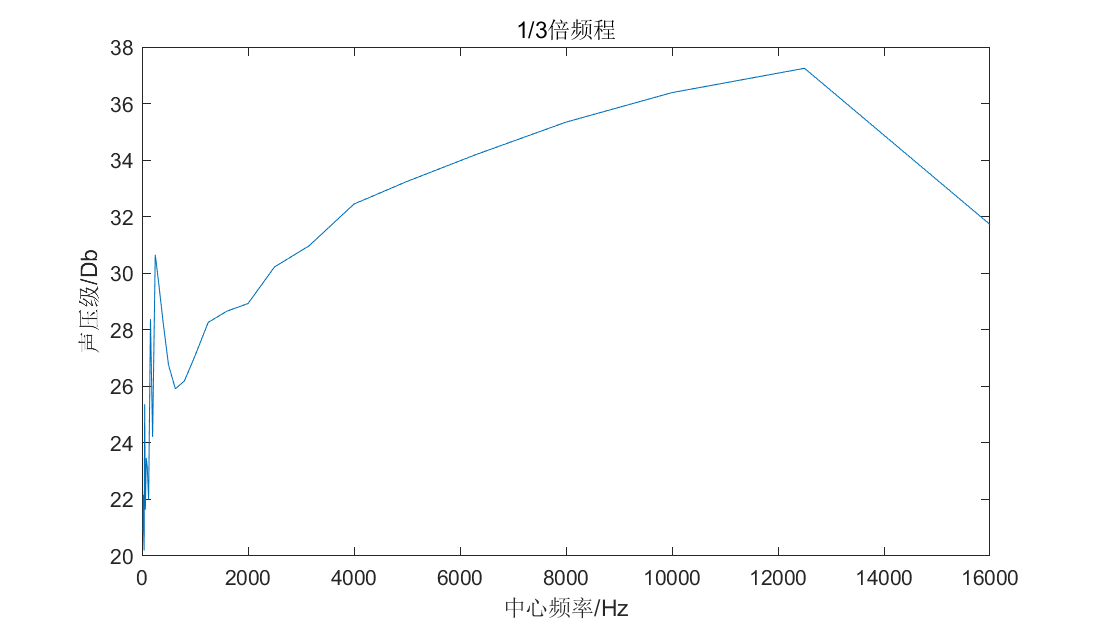• ## 三分之一倍频程谱

千次阅读 2019-10-26 18:59:55
三分之一倍频程谱是一种频率分析方法，它具有谱线少频带宽的特点。 倍频程实际上是频域分析中频率的一种相对尺度。倍频程谱是由一系列频率点以及对应这些频率点附近的频带内信号的平均幅值（有效值）所构成。这些...
三分之一倍频程谱是一种频率分析方法，它具有谱线少频带宽的特点。
倍频程实际上是频域分析中频率的一种相对尺度。倍频程谱是由一系列频率点以及对应这些频率点附近的频带内信号的平均幅值（有效值）所构成。这些频率点称为中心频率fc，中心频率附近的频带出于下限频率fl和上限频率fu之间。
三分之一倍频程谱是按逐级式频率进行分析的，它是由多个带通滤波器并联组成，为的是使这些带通滤波器的带宽覆盖整个分析频带。根据国籍电工委员会（IEC）的推荐，三分之一倍频程的中心频率为：
fc = 1000*10^(3n/30)Hz (n = ...-3,-2,-1,0,1,2,3...)
但在实际应用中，通常采用中心频率是其近似值。按照我国现行标准规定，中心频率为1Hz，1.25Hz，1.6Hz，2Hz，2.5Hz，3.15Hz，4Hz，5Hz，6.3Hz，8Hz，10Hz，...。可以看出，每隔三个中心频率，频率值增加一倍。三分之一倍频程的上下限频率以及中心频率之间的关系为：
fu/fl = 2^(1/3);fc/fl = 2^(1/6);fu/fc = 2^(1/6)
三分之一倍频程带宽为
△f = fu - fl
对于三分之一倍频程谱可以通过两种处理方法得到。一种方法是在整个分析频率从范围，按照不同的中心频率从定义对采样信号进行带通滤波，然后，计算出滤波后数据的均方值或均方根值（有效值），这样，变得到对应每个中心频率的功率谱值或幅值谱值。由于但分之一倍频程谱的滤波带与中心频率的比值是不变的，这种处理方法称为恒定百分比带宽滤波法。另一种方法首先对采样信号进行快速傅立叶变换，计算出功率谱或幅值谱，然后用功率谱或幅值谱的数据，计算每一个中心频率带宽内数矩的平均值，这样便处理得到三分之一倍频程谱值。这种方法显然要比第一种方法处理效率要高的多。
%%%%%%%%%%%%%%%%%%%%%%%%%%%%%%%%%%%%%%%%%%%%%%%%%%%%%%%%%%%%%%%%%%%%%%%%%%% % 三分之一倍频程处理 %%%%%%%%%%%%%%%%%%%%%%%%%%%%%%%%%%%%%%%%%%%%%%%%%%%%%%%%%%%%%%%%%%%%%%%%%%% clear              % Remove items from workspace, freeing up system memory clc                % Clear Command Window clf                % Clear current figure window close all hidden   % removal draws only those lines that are not obscured                    % by other objects in the field of view. format long %%%%%%%%%%%%%%%%%%%%%%%%%%%%%%%%%%%%%%%%%%%%%%%%%%%%%%%%%%%%%%%%%%%%%%%%%%% % 加载数据 fun = @(t) sin(50*2*pi*t) +sin(20*2*pi*t) + randn(size(t)); sf = 300;                       % 采样频率 n = 1000;                      % 数据长度 t = 0:1/sf:(n-1)/sf;            % 建立离散时间列向量 x = fun(t);                     % 产生时间序列数据
% 定义三分之一倍频程的中心频率 f = [1.00 1.25 1.60 2.00 2.50 3.15 4.00 5.00 6.300 8.00]; fc = [f,10*f,100*f,1000*f,10000*f]; oc6 = 2^(1/6);        % 中心频率与下限频率的比值 nc = length(fc);      % 取中心频率总的长度      nfft = 2^nextpow2(n); % 大于并最接近n的2的幂次方长度 a = fft(x,nfft);      % FFT变换 yc = zeros(1,nc); for j = 1:nc          fl = fc(j)/oc6;  % 下限频率          fu = fc(j)*oc6;      % 上限频率       nl = round(fl*nfft/sf+1);  % 下限频率对应的序号       nu = round(fu*nfft/sf+1);  % 上限频率对应的序号       if fu > sf/2     % 如果上限频率大于折叠频率则循环中断          m = j-1; break       end     % 以每个中心频率段为通带进行带通频域滤波        b = zeros(1,nfft);       b(nl:nu) = a(nl:nu);       b(nfft-nu+1:nfft-nl+1) = a(nfft-nu+1:nfft-nl+1);       c = ifft(b,nfft);     %计算对应每个中心频率段的有效值       yc(j) = sqrt(var(real(b(1:n)))); end %绘制输入时程曲线图形 subplot(2,1,1);plot(t,x); xlabel('时间　(s)');ylabel('加速度 (g)');grid on;                      %绘制三分之一倍频程有效值图形 subplot(2,1,2); plot(fc(1:m),yc(1:m)); xlabel('频率 (Hz)');ylabel('有效值'); grid on;
展开全文数字信号处理
• 根据流量数据服务公司StatCounter的统计，Chrome已经据全球浏览器市场三分之一的份额了，而且增长的势头不减。（参阅下面的表格） 根据StatCounter的数据，2012年7月份，Chrome在全球市场份额已经达到33.8%，同年...还记得在五月份，Chrome浏览器曾经超越IE成为世界第一浏览器长达一周的时间吗？后来有整整一个月都超越了。
根据流量数据服务公司StatCounter的统计，Chrome已经据全球浏览器市场三分之一的份额了，而且增长的势头不减。（参阅下面的表格）
根据StatCounter的数据，2012年7月份，Chrome在全球市场份额已经达到33.8%，同年六月份是32.8%，去年7月份是22.1%。
IE浏览器仍然坚挺地占据32%的份额，而Firefox正持续减少，现在只有23.7%的份额了。苹果的Safari以7.1%的份额占据第四位。值得一提的是Chrome浏览器上个月在欧洲地区已经占据头把交椅，第一次超过Firefox。到目前为止，Chrome在南美洲和亚洲是被使用最频繁的浏览器，而在美国，英国和澳大利亚，人们更习惯用IE。
背景资料：Chrome浏览器仅仅是4年前开始开发的。
StatCounter的数据可靠性虽然一直受到质疑，但它的报告还是被看做评价浏览器的标准。当然他们的数据不可能百分百准确，但是在总体趋势上一般是准确的。
StatCounter的数据来源于超过150亿个网页和超过300个使用了他们的统计插件的网站（他们不像Net Applications只统计独立IP的行为）。
你用的是什么浏览器呢，为什么？传智播客收集整理，关注java培训，php培训，提供java入门教程、java程序设计教程、java视频教程下载


展开全文浏览器 chrome firefox java safari
• 1. 相关概念介绍 ...这里提供能谱和三分之一倍频程能谱相应的代码： clc clear close all %% 实验 % xn(:,1) = 1:320000; % fs = 32000; % 采样频率 % ts = 1/fs; % 取样时间 % L = size(xn,1); % 总取样次
1. 相关概念介绍
其频谱、能谱、功率谱、倍频程谱的相关概念可看这篇博客：https://blog.csdn.net/liyuanbhu/article/details/42675765
2. 代码实现
这里提供能谱和三分之一倍频程能谱相应的代码（代码不易，请点赞+收藏）：
clc
clear
close all
%% 实验
% xn(:,1) = 1:320000;
% fs = 32000;      % 采样频率
% ts = 1/fs;     % 取样时间
% L = size(xn,1);     % 总取样次数
% t = (0:L-1) * ts;
% xn(:,2) = sin(2*pi*1000*t) + sin(2*pi*5000*t);

%% 正文

% 第一列为采样时刻，第二列为采样数值

%%%%%%%%%%%%%%%%%% 快速傅里叶变换 %%%%%%%%%%%%%%%%%%%%%

fs = 32000;      % 采样频率
ts = 1/fs;     % 取样时间
L = size(xn,1);     % 总取样次数
t = (0:L-1) * ts;
x = xn(:,2);
x = detrend(x,0);

%% 傅里叶变换
nfft = 2^15;

Y1 = fft(x,nfft);     % 傅里叶变换

f1 = (0:nfft-1)/(nfft-1)*fs;      % 频率向量

f = f1(1:nfft/2);       % 根据对称性取半

Y = Y1(1:nfft/2)*2/nfft;   % 根据对称性取半

YE = abs(Y); % 频域中的能量

ji_Ya = 2*10^(-5);  % 声压级基底

%% 计算频谱声压级
Ya_1 = 20 * log10(YE/ji_Ya);  % 计算频谱声压级

%% 计算总声压级
% 找出10-8k
YE_z = YE(find(f>=10&f<8000));
Z_Ya = 20 * log10(sqrt(sum(YE_z.^2))/ji_Ya)-3

%% 三分之一倍频程
% 定义三分之一倍频程的中心频率fc
fc = [20 25 31.5 40 50 63 80 100 125 160 200 ...
250 315 400 500 630 800 1000 1250 1600 2000 ...
2500 3150 4000 5000 6300 8000 10000 12500 16000];
% 下限频率
fl = round(fc/(2^(1/6)));
% 上限频率
fu = round(fc*(2^(1/6)));

fu(end) = f(end);   % 修复fu，末尾变为16000

%%
% 频率向量f中有L/2个数据，对应的频率是(0：L/2-1)/(L/2-1)*fs/2;
nl = round(fl*2/fs*(nfft/2-1) + 1); % 下限频率对应的频率向量的序号
nu = round(fu*2/fs*(nfft/2-1) + 1); % 上限频率对应的频率向量的序号
nc = length(fc); % 中心频率的长度

for i = 1:nc
nn = zeros(1,nfft);
nn(nl(i):nu(i)) = Y1(nl(i):nu(i));
nn(end-nu(i)+1:end-nl(i)+1) = Y1(end-nu(i)+1:end-nl(i)+1);
cc = ifft(nn);
YE_C(i) = sqrt(var(real(cc(1:nfft))));     % 求取1/3倍频程
%     YE_C(i) = sqrt(sum(YE(nl(i):nu(i)).^2)/2);    % 求取第i个中心频率的能量：频带的平均能量；
end

Ya_2 = 20 * log10(YE_C/ji_Ya); % 计算中心频率的声压级

%% 画图
a(1) = figure(1);
set(gca,'FontSize',10);
plot(0:length(t)-1,x);
title('原始信号');
xlabel('采样序号/采样频率为32000Hz');
ylabel('x');
set(gcf,'position',[100,100, 700, 400]); %设定figure的位置和大小 get current figure
set(gcf,'color','white'); %设定figure的背景颜色

a(2) = figure(2);
plot(f,Ya_1);
set(gca,'FontSize',10);
title('频谱');
xlabel('频率/Hz');
ylabel('声压级/Db');
set(gcf,'position',[100,100, 700, 400]); %设定figure的位置和大小 get current figure
set(gcf,'color','white'); %设定figure的背景颜色

a(3) = figure(3);
set(gcf,'color','white'); %设定figure的背景颜色
set(gca,'FontSize',10);
plot(fc,Ya_2);
title('1/3倍频程');
xlabel('中心频率/Hz');
ylabel('声压级/Db');
set(gcf,'position',[100,100, 700, 400]); %设定figure的位置和大小 get current figure

%% 导出数据
write_all = zeros(L,6);
write_all(:,1:2) = xn;
write_all(1:nfft/2,3) = f;
write_all(1:nfft/2,4) = Ya_1;
write_all(1:nc,5) = fc;
write_all(1:nc,6) = Ya_2;

xlswrite('matlab实验结果.xlsx',write_all);

saveas(a(1),'原始信号.bmp');
saveas(a(2),'频谱.bmp');
saveas(a(3),'三分之一倍频程.bmp');

3. 场景仿真：
输入数据：得到的能谱得到的三分之一倍频程能谱展开全文信号处理 数字信号处理 matlab 傅立叶分析 机器学习
• //============================================================================ // Name : 100题数组中出现次数超过一半的数字.cpp // Author : // Version : // Copyright : Your copyright notice // Descr
//============================================================================
// Name        : 100题之数组中出现次数超过一半的数字.cpp
// Author      :
// Version     :
// Description : Hello World in C++, Ansi-style
//============================================================================
// 数组中超过出现次数超过一半的数字以及超过三分之一的两个数字，超过四分之一的三个数字

#include <iostream>
using namespace std;
const int MAX = 65535;

void MoreThanForthNum(int *a,int length,int &first,int &second,int &thrid)
{
if( !a || length <= 0 )
throw new string("no valid input");

if( length <= 2 )
throw new string("no valid input");

if( length == 3 )
{
first = a;
second = a;
thrid = a;
return ;
}

first = 0;
second = 0;
thrid = 0;
int symbol = a, number = 1;
int symbol1 = 0, number1 = 0;
int symbol2 = 0, number2 = 0;

for( int i = 1;i < length;i++ )
{
if( !number && symbol1 != a[i] && symbol2 != a[i] )
{
symbol = a[i];
number = 1;
}
else if( symbol == a[i] )
{
number++;
}
else if( symbol1 != a[i] && symbol2 != a[i] )
{
number--;
}

if( !number1 && symbol != a[i] && symbol2 != a[i] )
{
symbol1 = a[i];
number1 = 1;
}
else if( symbol1 == a[i] )
{
number1++;
}
else if( symbol != a[i] && symbol2 != a[i] )
{
number1--;
}

if( !number2 && symbol != a[i] && symbol1 != a[i] )
{
symbol2=a[i];
number2=1;
}
else if( symbol2 == a[i] )
{
number2++;
}
else if( symbol != a[i] && symbol1 != a[i] )
{
number2--;
}
}

first = symbol;
second = symbol1;
thrid = symbol2;
number1 = 0;
number = 0;
number2 = 0;

for(int i = 0; i < length; i++)
{
if( a[i] == first )
{
number++;
}

if( a[i] == second )
{
number1++;
}

if( a[i] == thrid )
{
number2++;
}

}

if( !( number * 4 >= length && number1 * 4 >= length && number2 * 4 >= length ) )
{
throw new string("invalid input");
}

return;
}

bool MoreThanThridNum(int *a,int length,int &first,int &second)
{
if( !a || length <= 0 )
throw new string("no valid input");
if( length == 1 )
throw new string("no valid input");

if( length == 2 )
{
first = a;
second = a;
return true;
}

int symbol = a, number = 1;
int symbol1 = MAX, number1 = 0;

for( int i = 1; i < length; i++ )
{
if( !number && symbol1!=a[i] )
{
symbol = a[i];
number = 1;
}
else  if( symbol == a[i] )
{
number++;
}
else
{
number--;
}

if( !number1 && symbol != a[i] )
{
symbol1 = a[i];
number1 = 1;
}
else if( !number1 && symbol == a[i] )
{
symbol1 = MAX;
continue;
}
else if( symbol1 == a[i] )
{
number1++;
}
else if( symbol1 != MAX )
{
number1--;
}

}

first = symbol;
second = symbol1;
return true;
}

int MoreThanHalfNum( int *a,int length )
{
if(!a||length<=0)
throw new string("no valid input");

int symbol = a;
int number = 1;

for(int i = 1; i < length; i++)
{
if(symbol == a[i])
number++;
else
number--;

if( number == 0)
{
symbol = a[i];
number = 1;
}
}

number = 0;

for(int i = 0; i < length; i++ )
{
if( symbol == a[i] )
number++;
}

if( number * 2 >= length )
return symbol;
else
throw new string("no valid input");

}

/*
//获取数组中出现次数超过数组长度1/3的元素, 该算法错误
bool getMoreThanThird( int a[], int low, int high, int &result )
{
bool ret = true;

if( NULL == a || low > high )
{
ret = false;
cout << "getMoreThanThird func: err -1, NULL==a || low > high" << endl;
return ret;
}

if( low == high || low + 1 == high )
{
result = a[low];
return ret;
}

int cnt = 2;
int value = a[low];

for( int i = low + 1; i <= high; ++i )
{
if( a[i] == value )
{
++cnt;
}
else
{
--cnt;

if( 0 == cnt )
{
cnt = 2;
value = a[i];
}
}
}

cnt = 0;

for( int i = low; i <= high; ++i )
{
if( a[i] == value )
{
++cnt;
}
}

if( cnt > ( high - low + 1 ) / 3 )
{
result = value;
}
else
{
cout << "getMoreThanThird func: err -1, no this element whose count is bigger than the length of array" << endl;
ret = false;
}

return ret;

}
*/

int main()
{
//int a={2,2,3,3,2,2,3};
int a[] = {5, 5, 5, 7, 7, 8, 8, 8, 8, 2,
2, 2, 2, 2, 2, 2, 2, 5, 5, 5,
6, 5, 6, 7, 7, 7, 7, 7, 7, 7,
7, 5, 7, 2, 2, 2, 5, 5, 5, 8};

int length=40;
//  cout << MoreThanHalfNum(a,length)<< endl;
int first,second,thrid;
//  MoreThanThridNum(a, length,first,second);
//  cout<<first<<" "<<second<<endl;
MoreThanForthNum(a, length,first,second,thrid);
cout<<first<<" "<<second<<" "<<thrid<<endl;

//getMoreThanThird( a, 0, 39, first );
cout << first << endl;
return 0;
}

展开全文• ## 二分答案法、三分法

万次阅读 多人点赞 2016-03-15 16:56:43
• 二分搜索与三分搜索的应用： 二分和三分都利用了分治的思想,都是通过不断缩小查找的范围,把问题分解为更小的子问题直到找到解为止，,二分的时间复杂度为log2（n）,而三分的时间复杂度为3log3(n)，两者都是非常高效...ACM学习 搜索
• //96单位,以这个长度\位置作为 音 ‘羽 6 la’ 有 //就有 三分去其乘，//96三等分每份32，即32*3=96，减去被乘数（三分之一），96-32=64 //译法2//三分后去掉4*8，（“乘”如果代指数字 ，应该去掉4个基本数八，...
• 三分屏课件全自动实时合成系统 ---- 三分屏课件制作工具王者 （China-DRM倾力推荐，国内最好用的三分屏课件制作工具----无需专业知识，做出最专业的课件！） 三分屏课件全自动实时合成系统简单易用，能够轻松...工具 ftp服务器 服务器 加密 web
• 以下是我这个系列的相关文章，有兴趣可以参考一下，可以给个喜欢或者关注我的文章。 [Android]如何做个崩溃率少于千分之三噶应用app（1）-module工程架构 ...[Android]如何做个崩溃率少于千分之三噶应用app（4android
• 三分频电路 module half_clk(reset, clk_in, clk_out); input reset, clk_in; output clk_out; reg clk_out; reg[1:0] cnt; always @(posedge clk_in or negedge clk_in) begin if(!reset) beg...分频器 Verilog 二分频
• ## 二分查找&三分查找

千次阅读 2019-07-12 21:37:11
三分查找 二分查找（折半查找）是查找算法中一种有效的算法：输入个有序序列（数组）和个元素，如果该元素包含在改有序序列中，则返回其位置，若不存在，则返回**-1**（或NULL）。 二分查找的原理：对于个...算法 C++
• 转贴地址：http://www.joxue.com/no02/pc/code/code03/200602/12676.html如果你是个Web开发者，应该也写过这种接受用户输入的JavaScript代码： <input type="text" name="age" onchange="retujavascript 浏览器 mozilla xhtml 语言
• 也许很多人已经看过，送给那些没看过这个贴子的人吧。 　 　台湾最大的外商银行——花旗，个卧虎藏龙的金融竞技场。 　花旗人的外表，多半光鲜亮丽、自信满满...进入花旗银行十年，黄毅从不加班，他只有次在周
•二分法
• 埃及分数 把一个分数分解成n个 m分之一的形式 【贪心算法】 设a、b为互质正整数，a 步骤一： 用b 除以a，得商数q1 及余数r1。（r1=b - a*q1） 步骤二：把a/b 记作：a/b=1/(q1+1）+(a-r)/b(q1+1） ...
• 其实还是一样的，只是这里要用到两个三分了，不仅仅满足第个要求，第二个要求也要一并给他满足了，接着这样的最值就是题目所要求的了。下面是搓搓的代码：   #include #include #include using ...算法 numbers output input distance
• 地图之一 新旧图幅介绍 最近工作中需要用到地图幅功能，包括根据经纬度比例尺计算新旧图幅号、由图幅号计算图幅角点坐标、新旧图幅号的转换等。就重新把地图幅的知识复习了一遍，发现网上有很多资料有...
• 二分、三分查找算法的原理及实现代码。 简单定义 在个单调有序的集合中查找元素，每次将集合分为左右两部分，判断解在哪个部分中并调整集合上下界，重复直到找到目标元素。 时间复杂度 O (logn)，优于...
• ﻿﻿ 衰分术曰：各置列衰，副并...不更得一鹿、三分鹿之一。簪裹得一鹿。上造得三分鹿之二。公士得三分鹿之一。 术曰：列置爵数，各自为衰，副并为法。以五鹿乘未并者，各自为实。实如法得一鹿。 〔二〕今有牛、
• DEM 维地形处理之一：创建维地形的源数据之地形高程DEM数据和纹理贴图数据 要创建一个地形，最基本的需要2个数据对象：  a.其中之一也是最重要的就是：地形的DEM高程数据。  b.其次，是纹理贴图数据...gdal osg
• 通过本篇的阅读你将学会了解Elastic-Job的定时时机,及如何通过片方式做个分布式的定时任务框架。了解常用的片策略，及如何自定义分布式片策略 目录 Elastic-Job如何通过SpringJobScheduler启动定时 ...
• ## 设计模式的三大分类及六大原则

万次阅读 多人点赞 2018-08-20 00:47:11
、设计模式的分类 总体来说设计模式分为大类： 创建型模式，共五种：工厂方法模式、抽象工厂模式、单例模式、建造者模式、原型模式。 结构型模式，共七种：适配器模式、装饰器模式、代理模式、外观模式、桥接...java 设计模式
• ，是种和前缀和相对的策略。 差概念 对于个数列 ，我们需要维护的数据是“相邻两个数差”。这种策略是，令，即相邻两数的差。我们称数列 为数列 的差分数列。 应用 它可以维护多次对序列的个区间...
• 设计占空比为50%的三分频电路 时序图工具 {signal: [ {name: ‘clk’, wave: ‘P…P…’}, {name: ‘clk1’, wave: ‘H.LH.LH.L’}, {name: ‘clk2’, wave: ‘lh.lh.lh.l’,phase:0.5}, {}, {name: ‘clk1...奇数分频 占空比50%
• 十、Python编程计算24点（之一） ----From a high school student's view to learn Python 关键字： python 高中生学编程 Python编程入门 计算24点 表达式计算 组合问题 一、问题介绍 对于1-9中任意取出的个...python 高中生学编程
• 数据库的范式为什么不清？不就那么几个范式吗，就晕了，缺氧了吧，窗户没有开，还是垃圾没有倒？ 有了这篇文章，就不信你还不清。数据库范式 的 来历 数据库的规范化（上篇博客有写到）的程度不同，便有了这么...数据库 算法
• 帧差法1. 什么是帧差法　原理,请点击下面的连接，进行了解： 帧差法 　说的通俗一点，这个流程就是：　1.1　结果1 = (第二幅图像 - 第幅图像) ∩ (第幅图像 - 第二幅图像) 　1.2　结果2 = 滤波...运动检测 行人检测 傅里叶变换 二分法
• 文章目录第范式定义以及分析:问题研究：第二范式必备知识点函数依赖：码：非主属性 ：定义分析：解决办法：问题研究：第范式：定义：分析：问题研究：BCNF范式分析问题研究小结： 第范式 定义以及分析: 首先是...第二范式 BCNF范式
• 前言：好久没有学数学了 前几天loli给高一的讲课涉及到了本章内容，所以来普及波 差序列 基本概念 ...是个序列，我们定义的（一阶）差...我们定义个序列的0阶差序列就是ta自己： 我们可以把个序...组合数学...